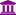# Why are the distributions in trees of Random Forests incorrect?

Member, University Professor Posts: 27University Professor
Im using a Random Forest to discover rules based on a simple dataset. After computing the model I check the trees to find leaves with a high confidence. when comparing the number of records shown by the tree description with the data in the dataset it turns out that the numbers are wrong. For instance, I have one attribute with a 50/50 distribution (greater than 0 and less than 0). The tree has the correct split value (0) but has 10 more records in the left branch.

Any ideas?

• Administrator, Moderator, Employee, RapidMiner Certified Analyst, RapidMiner Certified Expert, University Professor Posts: 3,078RM Data Scientist
Solution Accepted
Hi,
can you maybe provide an example for this?

Keep in mind that a Random Forest works on a bootstrapped set of the original data set, this may explain deviations.

Best,
Martin
- Head of Data Science Services at RapidMiner -
Dortmund, Germany
• Administrator, Moderator, Employee, RapidMiner Certified Analyst, RapidMiner Certified Expert, University Professor Posts: 3,078RM Data Scientist
edited February 24 Solution Accepted
of course. There are usually two factors, which make a Random Forest random.

First each node is only 'seeing' a subset of all attributes and than taking the best split in them.

Second, each tree is trained not on the full data set, but on 90% of the original data set. This 90% is not a random sample, but it is a bootstrapped sample. This means examples can be taken twice or even three times. (Ziehen mit Zurücklegen).
Have a look at the following process, which generates a  forest, which only consists of "root nodes". You can see that each root node as different distributions of yes/no. This is because of this bootstrapping.

<?xml version="1.0" encoding="UTF-8"?><process version="9.8.001">
<context>
<input/>
<output/>
<macros/>
</context>
<operator activated="true" class="process" compatibility="9.8.001" expanded="true" name="Process">
<parameter key="logverbosity" value="init"/>
<parameter key="random_seed" value="2001"/>
<parameter key="send_mail" value="never"/>
<parameter key="process_duration_for_mail" value="30"/>
<parameter key="encoding" value="SYSTEM"/>
<process expanded="true">
<operator activated="true" class="retrieve" compatibility="9.8.001" expanded="true" height="68" name="Retrieve Golf" width="90" x="179" y="34">
<parameter key="repository_entry" value="//Samples/data/Golf"/>
</operator>
<operator activated="true" class="concurrency:parallel_random_forest" compatibility="9.8.001" expanded="true" height="103" name="Random Forest" width="90" x="313" y="34">
<parameter key="number_of_trees" value="10"/>
<parameter key="criterion" value="gain_ratio"/>
<parameter key="maximal_depth" value="1"/>
<parameter key="apply_pruning" value="false"/>
<parameter key="confidence" value="0.1"/>
<parameter key="apply_prepruning" value="false"/>
<parameter key="minimal_gain" value="0.01"/>
<parameter key="minimal_leaf_size" value="2"/>
<parameter key="minimal_size_for_split" value="4"/>
<parameter key="number_of_prepruning_alternatives" value="3"/>
<parameter key="random_splits" value="false"/>
<parameter key="guess_subset_ratio" value="true"/>
<parameter key="subset_ratio" value="0.2"/>
<parameter key="voting_strategy" value="confidence vote"/>
<parameter key="use_local_random_seed" value="false"/>
<parameter key="local_random_seed" value="1992"/>
<parameter key="enable_parallel_execution" value="true"/>
</operator>
<connect from_op="Retrieve Golf" from_port="output" to_op="Random Forest" to_port="training set"/>
<connect from_op="Random Forest" from_port="model" to_port="result 1"/>
<portSpacing port="source_input 1" spacing="0"/>
<portSpacing port="sink_result 1" spacing="0"/>
<portSpacing port="sink_result 2" spacing="0"/>
</process>
</operator>
</process>

Best,
Martin

- Head of Data Science Services at RapidMiner -
Dortmund, Germany

• Member, University Professor Posts: 27University Professor
• Member, University Professor Posts: 27University Professor